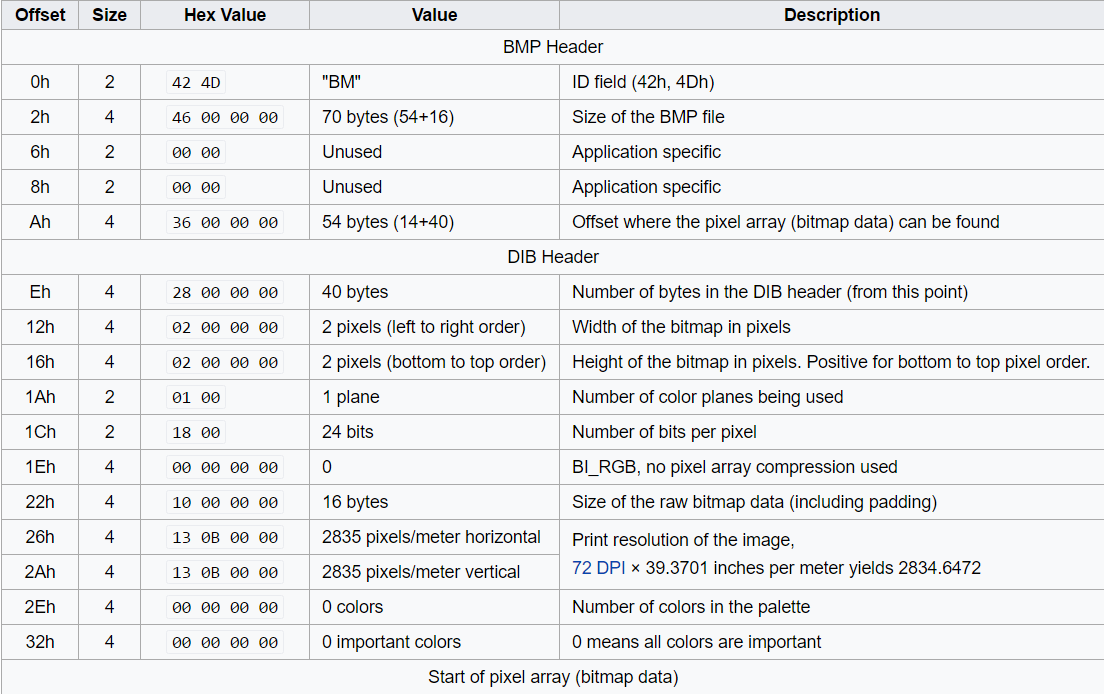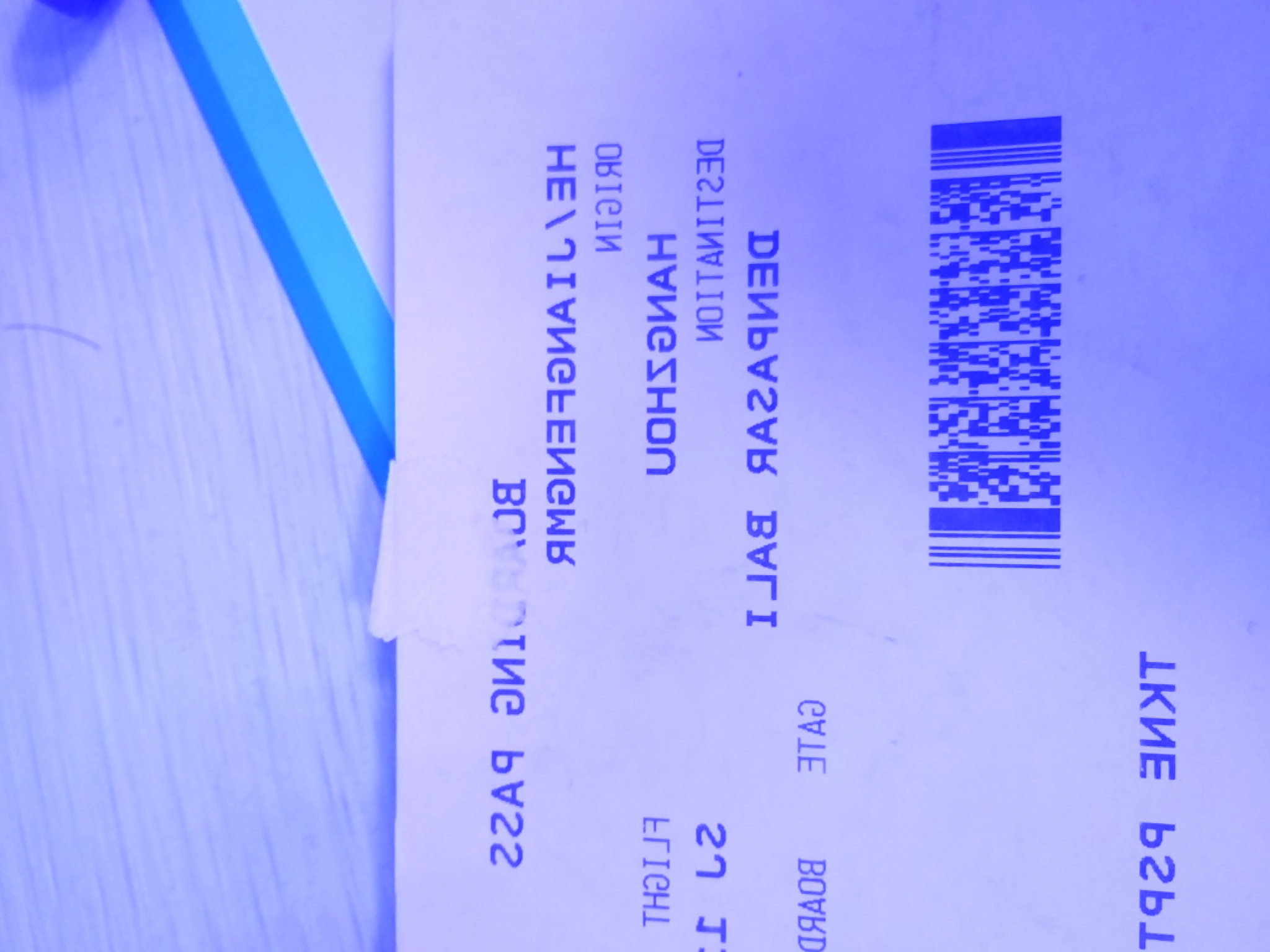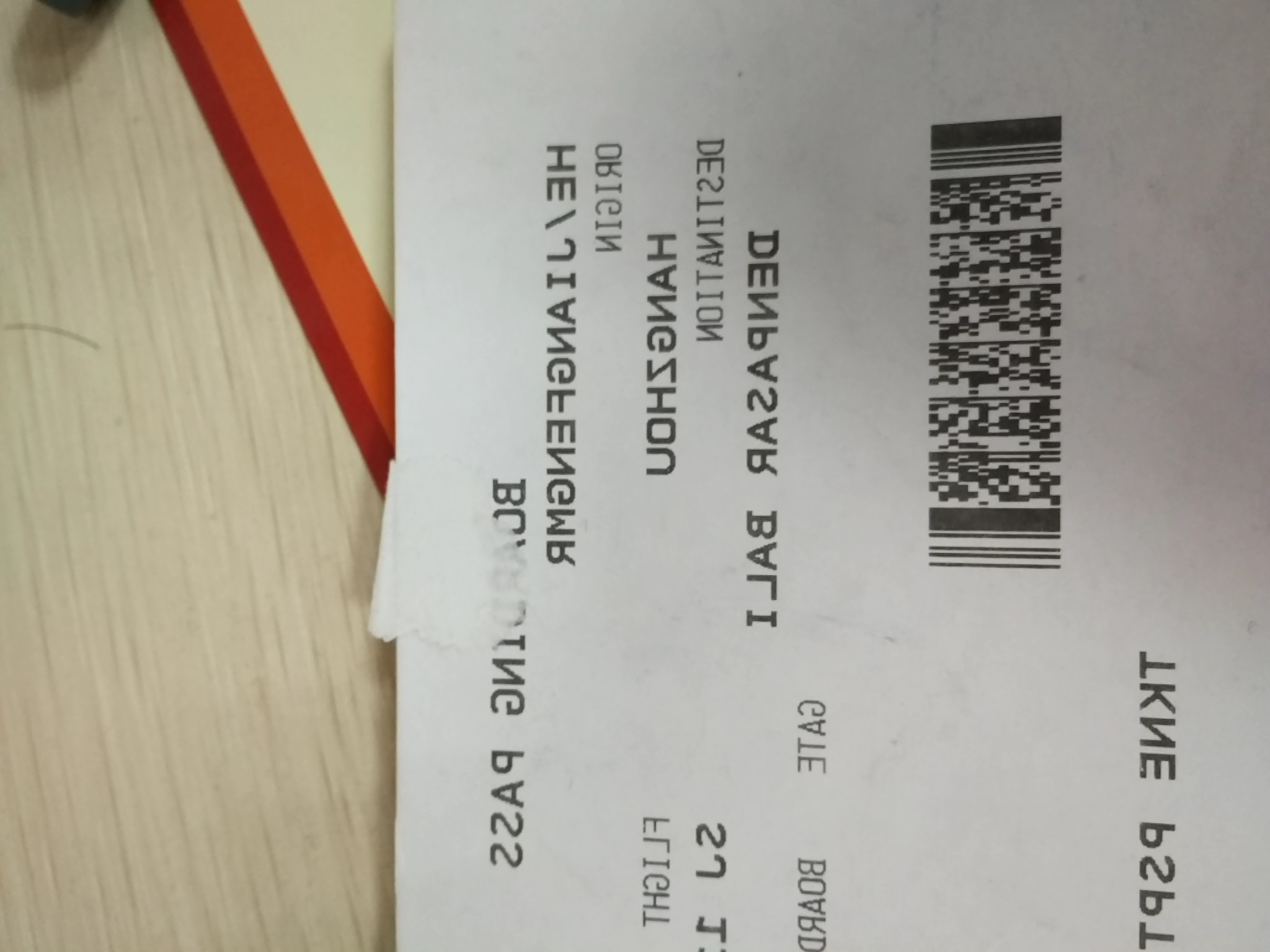×

# How to Convert NV21 Data to BMP File in Java

NV21 is the default image format used by Android camera. Assume you want to save the data and view it as a BMP file on PC, how to write code in Java without Android image APIs? Let’s do it from scratch.

## What is NV21

NV21 is a kind of YUV (also be referred to as YCbCr) format used for images. It has one luminance plane Y and one plane with V and U values interleaved. For a 2x2 group of pixels, you have 4 Y samples and 1 V and 1 U sample:

``````YYYYYYYY VUVU
``````

## NV21 to RGB

Get Y, V and U values from a byte array:

``````int total = width * height;
int[] rgb = new int[total];
int Y, Cb = 0, Cr = 0, index = 0;
int R, G, B;

for (int y = 0; y < height; y++) {
for (int x = 0; x < width; x++) {
Y = yuv[y * width + x];
if (Y < 0) Y += 255;

if ((x & 1) == 0) {
Cr = yuv[(y >> 1) * (width) + x + total];
Cb = yuv[(y >> 1) * (width) + x + total + 1];

if (Cb < 0) Cb += 127; else Cb -= 128;
if (Cr < 0) Cr += 127; else Cr -= 128;
}

}
}
``````

Convert YUV to RGB with the formula:

``````R = Y + Cr + (Cr >> 2) + (Cr >> 3) + (Cr >> 5);
G = Y - (Cb >> 2) + (Cb >> 4) + (Cb >> 5) - (Cr >> 1) + (Cr >> 3) + (Cr >> 4) + (Cr >> 5);
B = Y + Cb + (Cb >> 1) + (Cb >> 2) + (Cb >> 6);

// Approximation
// R = (int) (Y + 1.40200 * Cr);
// G = (int) (Y - 0.34414 * Cb - 0.71414 * Cr);
// B = (int) (Y + 1.77200 * Cb);
if (R < 0) R = 0; else if (R > 255) R = 255;
if (G < 0) G = 0; else if (G > 255) G = 255;
if (B < 0) B = 0; else if (B > 255) B = 255;

rgb[index++] = 0xff000000 + (R << 16) + (G << 8) + B;
``````

## What is BMP

BMP format is a raster graphics image format used to store bitmap digital images. It mainly consists of BMP header, DIB (device-independent bitmap) header and a pixel array. Here is an example of a 2×2 pixel, a 24-bit bitmap with pixel format RGB24 from Wikipedia:## How to Write Byte Array to BMP File

To save RGB array to an image, you can simply use Java Class BufferedImage and ImageIO:

``````BufferedImage bufferedImage = new BufferedImage(width, height, BufferedImage.TYPE_3BYTE_BGR);
bufferedImage.setRGB(0, 0, width, height, data, 0, width);
try {
ImageIO.write(bufferedImage, "JPG", new File(fileName));
} catch (IOException e) {
// TODO Auto-generated catch block
e.printStackTrace();
}
``````

However, it is more interesting to implement a custom Class yourself. We can construct a BMP Class as follows according to the BMP file structure and DIB structure:

``````public class BMP {

private byte[] id = { 0x42, 0x4D };
private int fileSize = 0;
private short spec1 = 0, spec2 = 0;
private int offset = 54;

private int biSize = 40;
private int biWidth, biHeight;
private short biPlanes = 0, biBitCount = 32;
private int biCompression, biSizeImage, biXPelsPerMeter, biYPelsPerMeter, biClrUsed, biClrImportant;

// bitmap data
private int[] data;

public BMP(int width, int height, short pixelBits, int[] pixels) {
biWidth = width;
biHeight = height;
biBitCount = pixelBits;
data = pixels;
fileSize = width * height * pixelBits / 8 + offset;
}

public int getFileSize() {
return fileSize;
}
}
``````

The BMP file stores integer values in little-endian format. Java uses big-endian format by default. Therefore, if you do not change the byte order, the generated BMP file cannot be correctly displayed. For example, if you use DataOutputStream to write bytes to a file:

``````DataOutputStream output;
try {
output = new DataOutputStream(new BufferedOutputStream(new FileOutputStream("big-endian.bmp")));
for (int i = 0; i < data.length; i++) {
output.writeInt(data[i]);
}
output.flush();
output.close();
} catch (FileNotFoundException e) {
// TODO Auto-generated catch block
e.printStackTrace();
} catch (IOException e) {
// TODO Auto-generated catch block
e.printStackTrace();
}
``````

View the image:To change the byte order, we can use ByteBuffer:

``````private byte[] getFile() {
ByteBuffer buffer = ByteBuffer.allocate(fileSize);
buffer.order(ByteOrder.LITTLE_ENDIAN);

buffer.put(id);
buffer.putInt(fileSize);
buffer.putShort(spec1);
buffer.putShort(spec2);
buffer.putInt(offset);

buffer.putInt(biSize);
buffer.putInt(biWidth);
buffer.putInt(biHeight);
buffer.putShort(biPlanes);
buffer.putShort(biBitCount);
buffer.putInt(biCompression);
buffer.putInt(biSizeImage);
buffer.putInt(biXPelsPerMeter);
buffer.putInt(biYPelsPerMeter);
buffer.putInt(biClrUsed);
buffer.putInt(biClrImportant);

for (int i = 0; i < data.length; i++) {
buffer.putInt(data[i]);
}

return buffer.array();
}
``````

Run the application again. Now the image displays well.## Source Code

https://github.com/yushulx/NV21-to-RGB